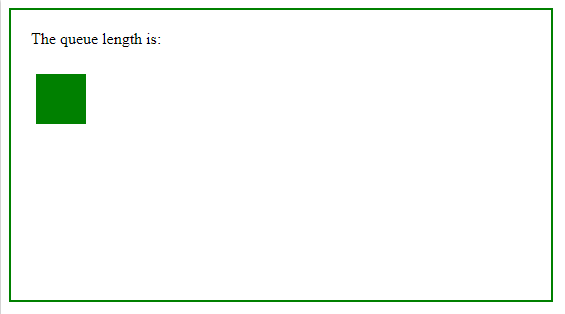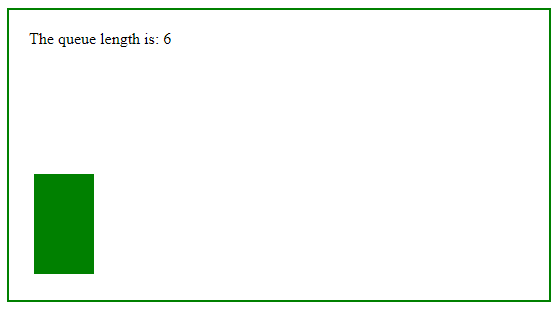Related Articles

# jQuery | queue() with Examples

• Last Updated : 13 Feb, 2019

The queue() method is an inbuilt method in jQuery which is used to show the queue of functions to be executed on the selected elements. In a queue one or more function wait for run.

• The queue() method can be used with the dequeue() method.
• An element may have several queues. Generally there is only one default jQuery queue.

Syntax:

`\$(selector).queue(queue_name)`

Parameters: This method accepts single parameter queue_name which is optional. It is used to set the queue name.

Below example illustrates the queue() method in jQuery:

Example:

 ```<``html``>``    ``<``head``>``        ``<``title``>The queue Method``        ``<``script` `src``=``        ``"https://ajax.googleapis.com/ajax/libs/jquery/3.3.1/jquery.min.js"``>``        ````         ` `        ````        ``<``script``>``            ``\$(document).ready(function() {``                ``\$("p").click(function() {``                    ``var div = \$("div");``      ` `                    ``div.animate({``                        ``left: "+=200"``                    ``}, 2000);``                    ``div.animate({``                        ``height: 200``                    ``}, "slow");``                    ``div.animate({``                        ``width: 150``                    ``}, "slow");``                    ``div.animate({``                        ``height: 100``                    ``}, "slow");``                    ``div.animate({``                        ``width: 60``                    ``}, "slow");``                    ``div.animate({``                        ``left: "-=200",``                        ``top: "+=100"``                    ``}, 2000);``      ` `                    ``\$("span").text(div.queue().length);``                ``});``            ``});``        ````        ``<``style``>``            ``div {``                ``width: 50px;``                ``height: 50px;``                ``position: absolute;``                ``left: 35px;``                ``margin-top: 10px;``                ``background-color: green;``            ``}``              ` `            ``body {``                ``width: 500px;``                ``height: 250px;``                ``border: 2px solid green;``                ``padding: 20px;``            ``}``        ````    ````      ` `    ``<``body``>``        ``<``p``>The queue length is: <``span``>``         ` `        ````        ``<``div``>``    `````

Output:
Before click on the paragraph element:After click on the paragraph element:My Personal Notes arrow_drop_up